\$B:#>,\$N0lGU(B

\$BKhF|!":#>,\$N0lGU\$r>e\$2\$F\$\$\$^\$9!#(B
\$B:#>,0{\$`\$b\$N\$K:\$\$C\$?\$i!";29M\$K\$7\$F\$_\$F\$/\$@\$5\$\$!#(B

2019\$BG/(B10\$B7n(B

 1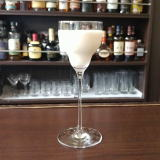\$B%3%s%5%s%W%7%g%s(B 2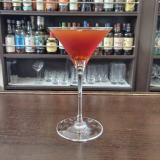(IL_WD0](B 3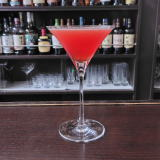(I@^L^Y%DWL^Y(B 4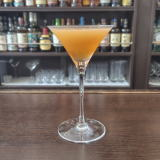(I4K_7-X1](B 5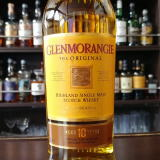(I8^Z]S0Z]<^(B 6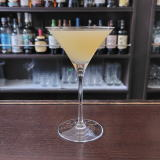(IM10%W2;^0(B 7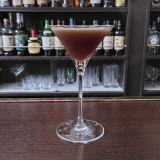(IL^W/8%O]C^0(B 8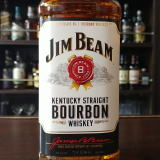(I<^Q%K^0Q(B 9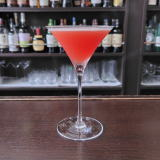(IRX0%K_/8L+0D^(B 10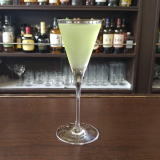(I@^0K^0(B 11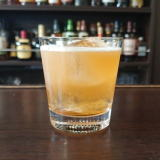(I[/8%[0S]D^(B 12 \$B5Y\$_(B 13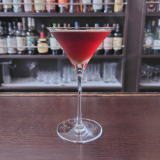(IA,0Y=(B 14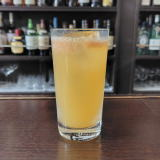\$B3A(IS0F(B 15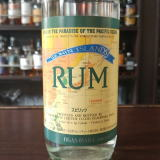\$B>.3^86%i%`(B 16(I70Y%[\2TY(B 17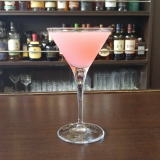(I8^Z0L_3^'2](B 18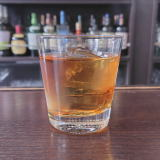(ILZ]A%3+YE/B(B 19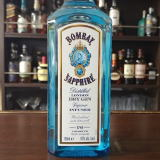(IN^]M^2%;L'21(B 20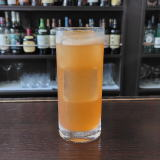(I50YD^O]%L(=^(B 21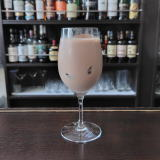(IS0B'YD%PY8(B 22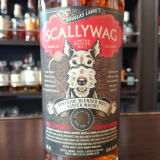(I=6X0\8^\$B!!(I6=8(B 23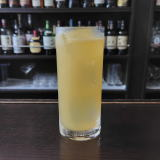(I5Z]<^%L(=^(B 24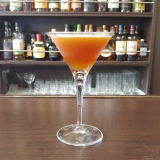(I6^>^/D(B 25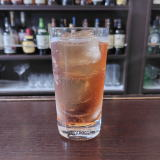(I<^]<^,0%<^]<^,0(B 26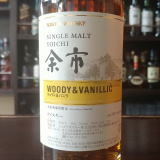\$BM>;T(I3/C^(\$B!u(IJ^FW(B 27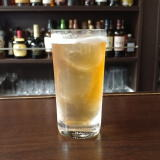(I=D0]%L*]=(B 28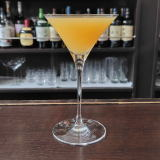(I5Z]<^%L^[/;Q(B 29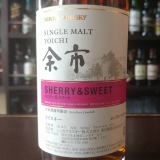\$BM>;T(I<*X0(B&(I=20D(B 30(IJ_X<^,](B 31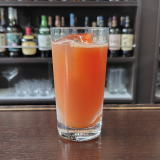(I=[0%D^W2J^0(B